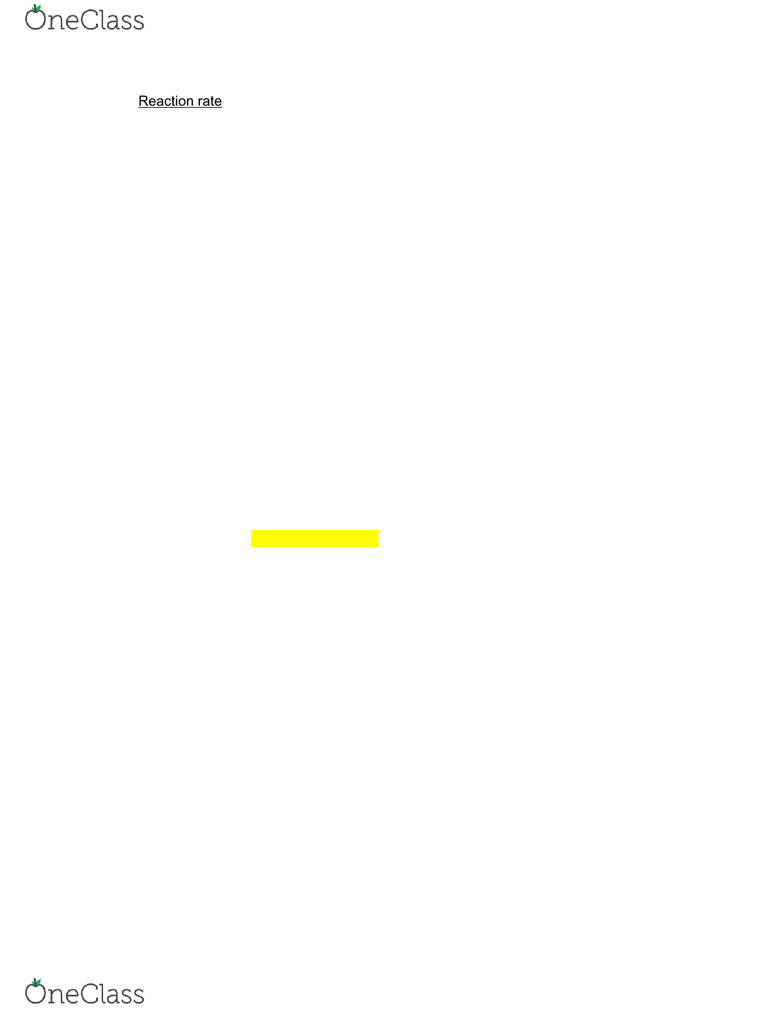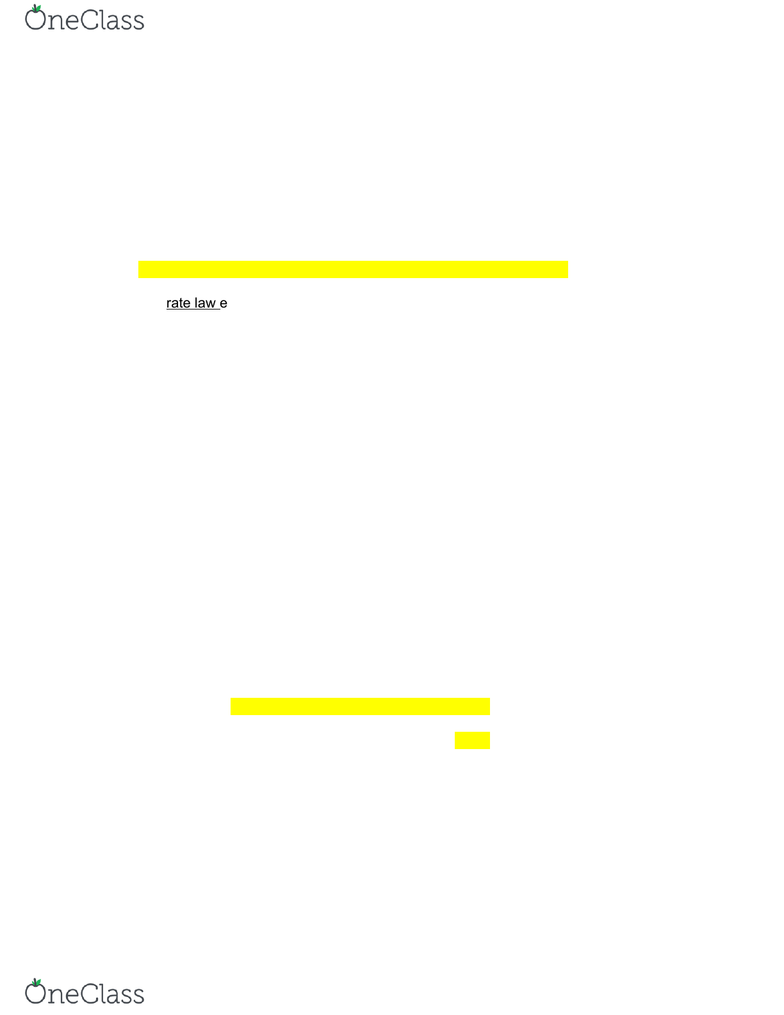# CHEM 1C Study Guide - Comprehensive Final Exam Guide - Oxygen, Equilibrium Constant, Rate Equation

86 views46 pages
School
UC-Irvine
Department
Chemistry
Course
CHEM 1C
ProfessorCHEM 1C
Unlock document

This preview shows pages 1-3 of the document.
Unlock all 46 pages and 3 million more documents.Unlock document

This preview shows pages 1-3 of the document.
Unlock all 46 pages and 3 million more documents.Reaction rate is the number (mol) of chemical reactions occuring in the system per
reaction volume (L) per time (s)
Reaction rate is usually measured in mol/(Lxs)
AB : reaction rate is the number of mol/(Lxs) of A converted into B every
second
Rate = -[A]/tchange in concentration of A over time period t
Rate = [B]/tchange in concentration of B over time period t
Because [A] decreases with time, [A] is negative
Average rate: ([A]final - [A]initial) / (tfinal - tinitial)
Example: concentration of O2 decreased from 0.0150 M to 0.0140 M in 3 minutes. Estimate the
average reaction rate
Average rate: –[O2]/t= –[O2]final – [O2]initial/ tfinal - tinitial
= –0.0140 M – 0.0150 M180 s
= 5.6 x 10-6 mol/ (Lxs)
Reaction rate = moles of reactions per volume (L) per time (s)
2 AB
For each mole of the reactions occurred:
Two moles of A disappeared
One mole of B was formed
To get the reaction rate, the rate of change in [A] and [B] has to be corrected by
the stoichiometric coefficients:
rate = -(½)( [A]/t) rate = [B]/t
find more resources at oneclass.com
find more resources at oneclass.com
Unlock document

This preview shows pages 1-3 of the document.
Unlock all 46 pages and 3 million more documents.In general, for reaction: aA + bB → cC + dD , the reaction rate can be calculated from
any of the concentrations:
Rate = - (1/a)([A]/t) = -(1/b)([B]/t) = (1/c)([C]/t) = (1/d)([D]/t)
Example: write the rate expression for the following reaction:
CH4(g) + 2O2(g) → CO2(g) + 2H2O(g)
Rate = - [CH4]/t = -(½)[O2]/t = [CO2]/t = - (½)[H2O]/t
The rate law expresses the relationship between the reaction rate and the
concentrations of the reactants
For the reaction: aA + bB → cC + dD , the rate law has the following form:
Rate = k[A]x[B]y
k is the reaction rate constant units: rate: M/s
Reaction is the xth order in A k,rate constant: M(1-x-y)/s
Reaction is the yth order in B
Reaction is (x + y)th order overall
The rate law does not include products
Example: what is the overall order of the following reaction, given the rate law?
2 X + 3 Y → 2 Z
Rate = k[X]1[Y]2
1 + 2 = 3, so the overall order is 3rd order
What is the unit constant of k from the previous example? 1/M2s
Rate laws are normally determined experimentally
Reaction order is usually defined in terms of reactant (not product) concentrations
The order of the reaction need not be related to the stoichiometric coefficient of the
reactant in the balanced equation
find more resources at oneclass.com
find more resources at oneclass.com
Unlock document

This preview shows pages 1-3 of the document.
Unlock all 46 pages and 3 million more documents.